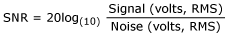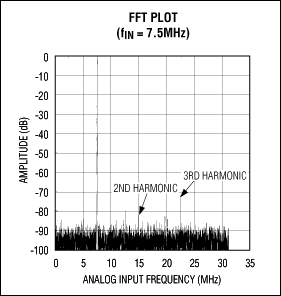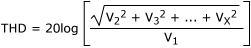# Data Conversion Calculator

Below is a list that describes what each conversion on the calculator means.

Decibel (dB) - A unit of relative amplitude defined on a logarithmic scale. For voltage values, dB is given by 20log(VA/VB). For power, it is 10log(PA/PB). When dB is referenced to a carrier signal, it is termed dBc; likewise, dB referenced to 1mW is termed dBm. For dBm, the load resistance must be known for the specification to determine the voltage or current equivalence (that is, 1mW into 50Ω).

Effective Number of Bits (ENOB) - The measured performance (in bits) of an analog-to-digital converter (ADC) with respect to the input frequency (fIN). As fIN increases, overall noise (particularly the distortion components) also increases, thereby reducing the ENOB and SINAD. See also signal-to-noise and distortion ratio (SINAD). ENOB is related to SINAD by the following equation:Resolution - When an analog signal is digitized, it is represented by a finite number of discrete voltage levels. The resolution is the number of discrete levels that are used to represent the signal. To more accurately replicate the analog signal, the resolution must be increased. Resolution is usually defined in bits. Using converters with higher resolutions will reduce the quantization error.

RMS - See root mean square (RMS).

Root Mean Square (RMS) - The effective value or effective DC value that an AC signal represents. For a sine wave, the RMS value is 0.707 times the peak value, or 0.354 times the peak-to-peak value.

SFDR - See spurious-free dynamic range (SFDR).

Signal-to-Noise and Distortion Ratio (SINAD) - The RMS value of the sine wave fIN (input sine wave for an ADC, reconstructed output sine wave for a ADC/DAC) to the RMS value of the noise of the converter from DC to the Nyquist frequency, including harmonic content. It is typically expressed in decibels. See also root mean square (RMS) and total harmonic distortion.Signal-to-Noise Ratio (SNR) - The RMS value of the sine wave fIN (input sine wave for an ADC, reconstructed output sine wave for a DAC) to the RMS value of the noise of the converter from DC to Nyquist frequency, excluding noise at DC and harmonic distortion content. It is typically expressed in decibels. See also root mean square (RMS).The ideal theoretical minimum conversion noise is caused by quantization noise error only and results directly from the data converters resolution.

(N): SNR = (6.02N +1.76)dB

Spurious-Free Dynamic Range (SFDR) - The ratio of the RMS value of the sine wave fIN (input sine wave for an ADC, reconstructed output sine wave for a DAC) to the RMS value of the peak spur observed in the frequency domain. It is typically expressed in decibels. SFDR is important in certain communication applications that require maximizing the dynamic range of the converter.Total Harmonic Distortion (THD) - The RMS value of the distortion appearing at multiples (harmonics) of the input (or output for a DAC) frequency to the RMS value of the input (or output) sine wave. Only harmonics within the Nyquist limit are included in the measurement. It is typically expressed in decibels:Here V2 to Vx are harmonics to the fundamental V1.